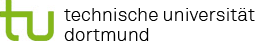You are here:

### Project R1: High-dimensional regression for screening of important genetic and environmental factors

In project R1, we will develop variable selection methods for regression models. The methods can be used to screen important genetic and environmental risk factors and their interactions. The study data considered here each have a very large number of genetic and other variables (p > 1 million). The studies NaKo, SALIA, and GINIplus have a relatively high number of observations with up to n=10,000 subjects, but this number is still well below the number of variables (n<<p). We will start analysing the NaKo data and use the results for validation on the other data sets, as the NaKo is currently the largest epidemiological study in Germany, with 200,000 subjects across different study centers. Since some targets such as disease outcomes are binary and others are continuous, e.g. blood pressure or lung function data, we will consider both logistic and linear regression models.

The main idea is to transfer our dimension reduction methods that are based on sampling and random projections from reducing observations to selecting variables. For linear models, a sketching approach based on subspace embeddings has been developed by Geppert et al. (2017) for linear regression with a small to moderate number p of variables. The aim is to extend this methodology and introduce a lasso penalty to use the method for screening genetic and environmental variables when the number of variables is very large. First approaches for reducing the number of variables were introduced by Nayebi et al. (2019) for linear regression using random projections and by Trippe et al. (2019) for generalised linear models employing low-rank approximations. For both approaches, a high degree of interpretability of the embedded variables is not ensured. Since maintaining interpretability is crucial in screening applications, we will formulate the above-mentioned approaches using sampling-based methods such as leverage scores, as suggested by Drineas et al. (2012) for linear regression.

Genetic markers are binary and, often, main effects are not the focus. Instead, the primary aim is to uncover interactions between the binary variables that affect the response. Methods such as logic regression are able to find combinations of the explanatory variables that capture higher-order relationships in the response. However, the number of explanatory variables that these methods can handle is limited. For both logistic and linear models one possibility is to reduce the number of variables prior to the analysis, e.g., by using cross-leverage scores and leverage scores as suggested in Parry et al. (2020). Sampling based on leverage or crossleverage scores will be transferred to a combination of genetic and environmental variables. In a second step, logic regression can be employed to select the most important variables and interactions in a logistic or linear regression setting. The sampling approaches should be employed directly on the data, before forming logic expressions, to preserve interpretability.

We will compare the approaches based on reducing the set of variables with component-wise boosting that is applied to the set of all variables. Component-wise boosting was developed by Binder et al. (2012) for case-control SNP data sets. This approach allows adapting different local logistic regression models for different groups of observations by integrating weights into component-wise boosting. Simultaneously, variables are selected and lead to models with few influence factors. We will extend this approach so that it can be applied to (a large number of) SNPs and environmental variables and their interactions.

The cluster-localised boosting approaches directly lead to subgroups of patients. While the projection approaches in general do not allow subgroup identification, the sampling-based approaches can be formulated to allocate observations to subgroups. A method to that end using leverage scores has been suggested by Gray and Ling (1984). Here, we will adapt this method to our setting.

#### References

• Binder H, Müller T, Schwender H, Golka K, Steffens M, Hengstler JG, Ickstadt K, Schumacher M (2012). Cluster-localized sparse logistic regression for SNP data. Statistical Applications in Genetics and Molecular Biology 11, 13, doi: 10.1515/1544-6115.1694.
• Drineas P, Magdon-Ismail M, Mahoney MW, Woodruff DP (2012). Fast approximation of matrix coherence and statistical leverage. J Machine Learning Research 13, 3475-3506, doi: 10.5555/2503308.2503352.
• Geppert L, Ickstadt K, Munteanu, A, Quedenfeld J, Sohler C (2017). Random projections for Bayesian regression. Statistics and Computing 27(1), doi: 10.1007/s11222-015-9608-z. Gray JB, Ling RF (1984). K-clustering as a detection tool for influential subsets in regression. Technometrics 26(4), doi: 10.1080/00401706.1984.10487980.
• Nayebi A, Munteanu A, Poloczek M (2019). A framework for Bayesian optimization in embedded subspaces. Proceedings of the 36th International Conference on Machine Learning, Long Beach, California, PMLR 97.
• Parry K, Geppert L, Munteanu A, Ickstadt K (2020). Cross-leverage scores for selecting subsets of explanatory variables. To appear in: J Computational and Graphical Statistics. Trippe B, Huggins J, Agrawal R, Broderick T (2019). LR-GLM: High-dimensional Bayesian inference using low-rank data approximations. Proceedings of the 36th International Conference on Machine Learning, Long Beach, California, PMLR 97.